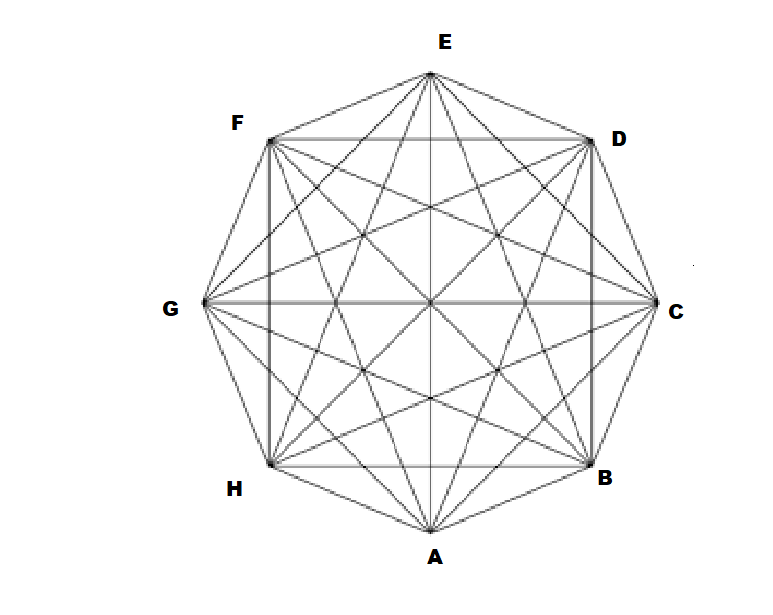QuestionAnswers

# The number of diagonals that can be drawn by joining the vertices of an octagon, isA) 28B) 48C) 20D) None of these

Hint: Here first we will find the number of lines that can be drawn through the vertices octagon and then find the number of diagonal of the polygon.
Octagon is 8 sided polygon which means it has 8 sides and 8 vertices.

Complete step by step solution:We know that the number of sides of an octagon is 8
Hence it has 8 vertices.
Therefore we need to find the number of lines that can be drawn from 8 points.
Now since we know that a line is drawn with the help of 2 points.
Therefore, we need to choose 2 points out of 8 points in order to draw a line.
Therefore,
The number of lines that can be drawn from 8 points is given by ${}^8C_2$lines
Now as we know that general formula for ${}^nC_r$ is:
${}^nC_r = \dfrac{{n!}}{{r!\left( {n - r} \right)!}}$
Where $n$ represents the number of items, and $r$ represents the number of items being chosen at a time.
Therefore applying this formula we get:
$\Rightarrow {}^8C_2 = \dfrac{{8!}}{{2!\left( {8 - 2} \right)!}}$
$\Rightarrow {}^8C_2 = \dfrac{{8!}}{{2! \times 6!}}$
On simplifying the above values,
$\Rightarrow {}^8C_2 = \dfrac{{8 \times 7 \times 6!}}{{6! \times 2 \times 1}}$
$\Rightarrow {}^8C_2 = \dfrac{{8 \times 7}}{2}$
On further simplifications, we get
$\Rightarrow {}^8C_2 = 4 \times 7$
$\Rightarrow {}^8C_2 = 28$
Hence 28 lines can be drawn with 8 points
Now since the octagon has 8 lines as its sides
Therefore we need to subtract 8 from total number of lines in order to get the number of diagonals
Hence the number of diagonals is given by:-
$Number{\text{ }}of{\text{ }}diagonals\; = \;Total{\text{ }}number{\text{ }}of{\text{ }}lines\; - \;total{\text{ }}number{\text{ }}of{\text{ }}sides{\text{ }}of{\text{ }}octagon$
Putting in the values we get:-
$\Rightarrow Number{\text{ }}of{\text{ }}diagonals\; = 28 - 8$
$\Rightarrow Number{\text{ }}of{\text{ }}diagonals\; = 20$

$\therefore$ The octagon has 20 diagonals. Hence, option C is the correct answer.

Note:
The octagon is a polygon in geometry, which has 8 sides and 8 angles.
That means the number of vertices is 8 and the number of edges is 8.
A regular octagon has all the sides and angles equal.
A diagonal is a line joining two opposite vertices of a polygon
So the student should consider only those lines which join two opposite vertices of the octagon to find the exact number of diagonals. The diagonals can also be calculated directly by sketching the figure of the octagon